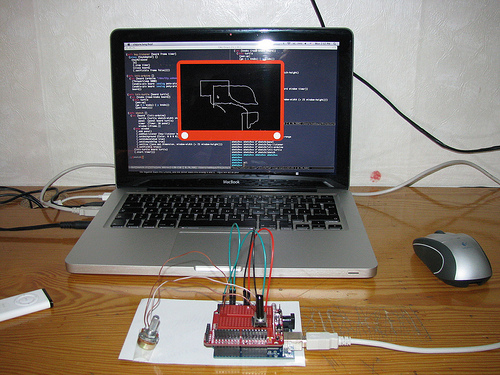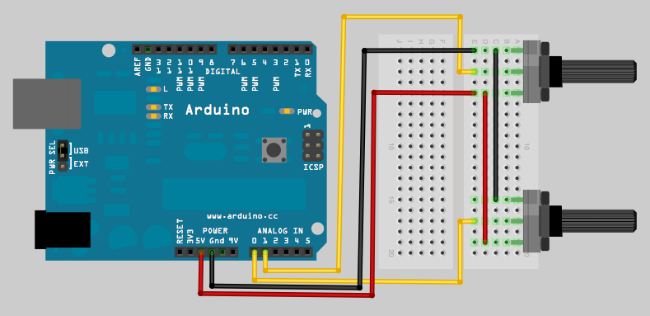## Etch A SketchA small Sunday hack inspired by the classical children's toy, for this project you need two potentiometers, connected to analog pins 0 and 1,Idea behind the project is simple, we read potentiometers, turn the values read into x and y coordinates for the turtle then command turtle to go to that coordinate.

(ns sketch
(:use turtle)
(:use clodiuno.core)
(:use clodiuno.firmata)
(:import (javax.swing JFrame JPanel)
(java.awt Color Rectangle)
(javax.swing Timer)
(java.awt.geom RoundRectangle2D$Double Ellipse2D$Double)))

(def potx-pin 0)
(def poty-pin 1)

(def window-width 640)
(def window-height 480)
(def sketch-width 600)
(def sketch-height 400)

;;WMath.cpp
(defn map-range [x in-min in-max out-min out-max]
(+ (/ (* (- x in-min) (- out-max out-min)) (- in-max in-min)) out-min))

x (int (map-range
potx 0 1024 (- (/ sketch-width 2)) (/ sketch-width 2)))
y (int (map-range
poty 0 1024 (- (/ sketch-height 2)) (/ sketch-height 2)))]
{:x x :y y}))


Potentiometers returns values between 0 and 1024, assuming our image is 600 by 400, we need to map these values between -300 to 300 on the x axis and -200 to 200 on the y axis, 0,0 being the center of the image.

(defn panel [board turtle]
(proxy [JPanel ActionListener] []
(paintComponent
[g]
(doto g
(.setColor Color/red)
(.fill (RoundRectangle2D$Double. 0 0 window-width window-height 60 60)) (.setColor Color/black) (.drawImage (:image @turtle) pad pad this) (.setColor Color/white) (.fill (Ellipse2D$Double. pad 430 40 40))
(.fill (Ellipse2D\$Double. (- sketch-width pad) 430 40 40))))
(actionPerformed
[e]
(go turtle (:x knobs) (:y knobs)))
(.repaint this))))


With every tick of the timer, we'll read the potentiometers, then command the turtle to goto that position and repaint the panel to reflect changes.

(defn key-listener [board frame timer]
(keyReleased
[e]
(.stop timer)
(close board)
(.setVisible frame false))))


When the sketch window receives a key event, we stop the timer, disconnect arduino and hide the window.

(defn init-arduino []
(let [board (arduino :firmata "/dev/tty.usbserial-A6008nhh")]
(enable-pin board :analog potx-pin)
(enable-pin board :analog poty-pin)
board))


By default pins aren't read, you need to tell arduino which ports you are interested, in this case we are interested in analog 0 and analog

(defn init-turtle [board turtle]
(doto turtle
(pen-up)
(go (:x knobs) (:y knobs))
(pen-down))))


Since we don't know the position of potentiometers when the application starts, we need to read the values once and position the turtle accordingly. When all the functions wired and timer started, you should have your Etch A Sketch.

(defn sketch []
(let [board  (init-arduino)
turtle (turtle sketch-width sketch-height)
panel  (panel board turtle)
timer  (Timer. 50 panel)
window (JFrame.)]
(doto window